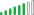cancel
Showing results for
Did you mean:Helper II

## Set value from existing column/row when adding new column to fact table.

I have a fact table which lists widgets, years, and an attribute of the widget in that year. For example:

Widget_ID    Year    Attribute
1001              2005       1
1001              2006       15
1001              2007       12
1001              2008       1

What I'd like to do is create another column that has the value of the widget's previous year attribute, which should look like this:

Widget_ID    Year    Attribute     PY Attribute
1001              2005        1                   N/A
1001              2006        15                 1
1001              2007        12                 15
1001              2008        1                   12

I've been playing around with CALCULATE and FILTER commands, but I feel very clumsy with DAX at this point. Here is the gist of what I've been trying:

PY Attribute = CALCULATE(MAX('Table'[Attribue]),'Table'[Year] = ('Table'[Year] - 1),'Table[Widget_ID] ='Table'[Widget_ID])

Any advice or tips would be appreciated.

1 ACCEPTED SOLUTIONHelper II

Yes, this worked; I used the MAXX option because my table did have more columns. The column formula turned out to be:

=MAXX(FILTER('Table',('Table'[Widget_ID] = EARLIEST('Table'[Widget_ID]) && 'Table'[Year] = EARLIER('Table'[Year])-1)),[Attribute])

2 REPLIES 2Solution Supplier

Is it what you were looking for?

```CALCULATE( MAX('Table'[Attribute])
,'Table'[Year] = EARLIER('Table'[Year])- 1
,'Table[Widget_ID] = EARLIER('Table'[Widget_ID])
)```

Note that, due to the way CALCULATE works, you may get unexpected results if your table has more columns than in your description.

You may prefer something like the following instead:

```MAXX( FILTER('Table'
,'Table'[Year] = EARLIER('Table'[Year])- 1
&& 'Table[Widget_ID] = EARLIER('Table'[Widget_ID]
)
, [Attribute]
)```Helper II

Yes, this worked; I used the MAXX option because my table did have more columns. The column formula turned out to be:

=MAXX(FILTER('Table',('Table'[Widget_ID] = EARLIEST('Table'[Widget_ID]) && 'Table'[Year] = EARLIER('Table'[Year])-1)),[Attribute])